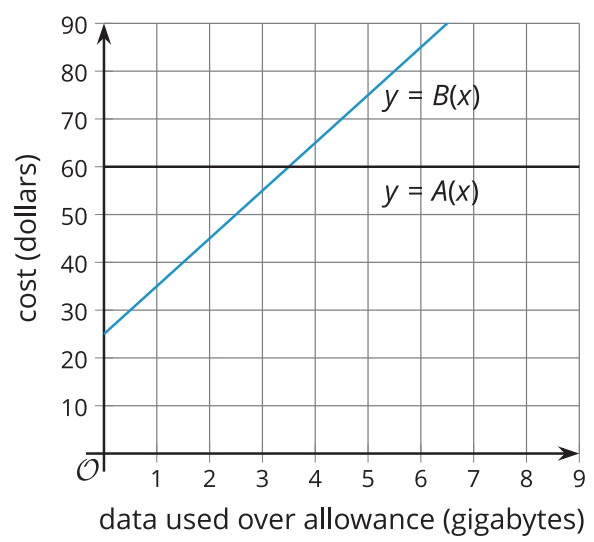# A.4.5.3 Function Notation and Graphing Technology

The function B is defined by the equation B(x)=10x+25. Use graphing technology to:

1. Find the value of each expression: B(6) B(2.75) B(1.482)

2. Solve each equation: B(x)=93 B(x)=42.1 B(x)=116.25

Refer back to the activity about data plans. Display the two graphs for all to see.Ask students to use the graphs to find:
• the values of A(0.8) and B(0.8)
• the solutions to A(x)=72 and B(x)=72
Next, display the two equations for all to see. (Hold off on discussing students’ responses.) Ask students to use the equations to find the same four values as they have just found using the graphs. A(x)=60 B(x)=10x+25 Invite students to compare and contrast the graphical and algebraic approaches for finding unknown inputs and outputs of linear functions. Discuss questions such as: “How easy was it to use the graph of B to find an output value such as B(0.8)? What about using the graph to find an input value, such as the x in B(x)=72?” (Both were fairly straightforward, but may not have been very precise. Some estimation was necessary. It was very easy to see that there was no solution to A(x)=72.) “How easy was it to use the rule B(x)=10x+25 to find an output value such as B(0.8)? What about finding an input value, such as the x in B(x)=72?” (Both were fairly simple, but if the rule or the given input or output involves numbers that are harder to compute by hand, it might be more complicated.) If students did the optional activity on using graphing technology, ask students to identify some ways that technology could help to find unknown input and output values of a function.